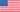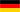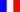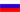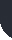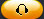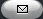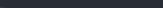Task Management Software for Team Collaboration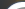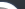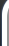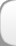HomeDownloadSupportPurchase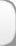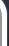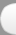Installation GuideStartUp GuideOnline Help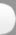# Accumulated Depreciation of Tangible Assets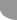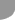Home  » Products  » VIP Task Manager Pro  » Tutorials  » Customization Guide  » Accumulated Depreciation of Tangible Assets

Accumulated Depreciation of Tangible Assets

Accumulated Depreciation of Tangible Assets is depreciation expense of companys assets accumulated over time. Tangible Assets are any companys resources that can be touched but are not sold to customers (such as equipment, vehicles, devices required to complete a project). Accumulated Depreciation of Tangible Assets can be calculated using different methods. Most of the companies use Straight Line Depreciation method because its the simplest method comparing with other methods for calculating Accumulated Depreciation (Double declining balance depreciation, Sum-of-the-years'-digits method). Under Straight Line Depreciation method depreciation charge is spread equally over all years of estimated useful life of asset.

Using Straight Line Depreciation method Accumulated Depreciation is calculated by the following formula:

Accumulated Depreciation = (Book Value Salvage Value) / Useful Life of Asset

Book Value is cost of tangible asset it was purchased at.

Useful Life of Asset is the estimated period of time within which tangible asset can be used before replacement.

Salvage Value (Residual Value or Scrap Value) is the estimated cost of the asset after its useful life. Sometimes salvage value is estimated as zero. In this case Accumulated Depreciation is calculated by the formula below:

Accumulated Depreciation = Book Value / Useful Life of Asset

To check Salvage Value of your assets you can consult financial companies that provide expertise on this topic.

If you need to calculate Accumulated Depreciation per Tangible Asset, you need to create task with the name of asset and then do the following:

• Add the custom field Book Value with code cf_book_value
•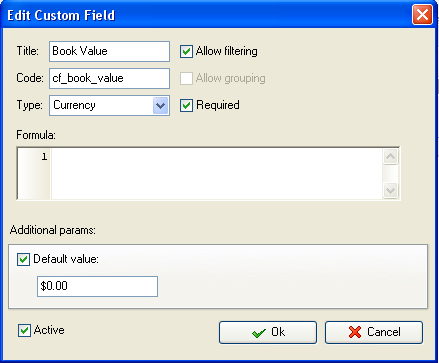• Add the custom field Salvage Value with code cf_salvage_life
•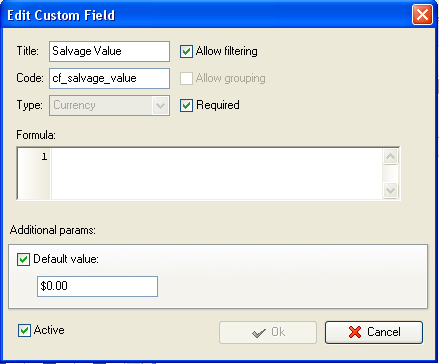• Add the custom field Useful Life with code cf_useful_life
•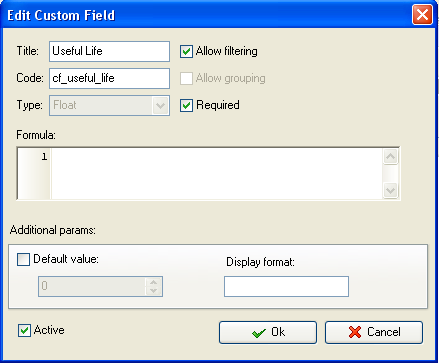• Add the custom field Accumulated Depreciation with the formula:
• (\$cf_book_value - \$cf_salvage_value) / \$cf_useful_life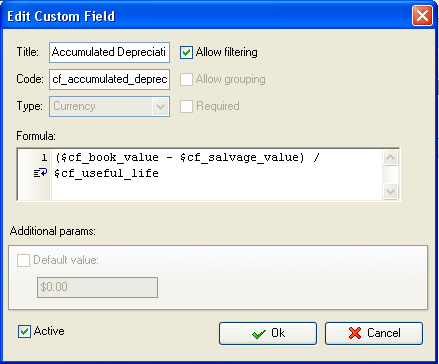Note: Add the values of the custom fields Book Value, Salvage Value and Useful Life for each task (or asset) and the program will automatically calculate Accumulated Depreciation for each asset you have.

• To enable the displaying of Accumulated Depreciation per task group or project select the root or parent task group and click Custom fields options
•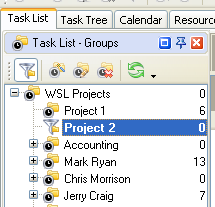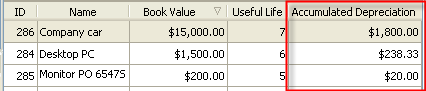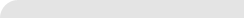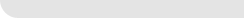» VIP Task Manager Pro VIP Task Manager Std Testimonials Turnkey Solution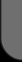ProductsSolutionsDownloadSupportPurchaseContactsForumVideoMy Account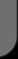Copyright © 2004 - 2023 Task Management Software